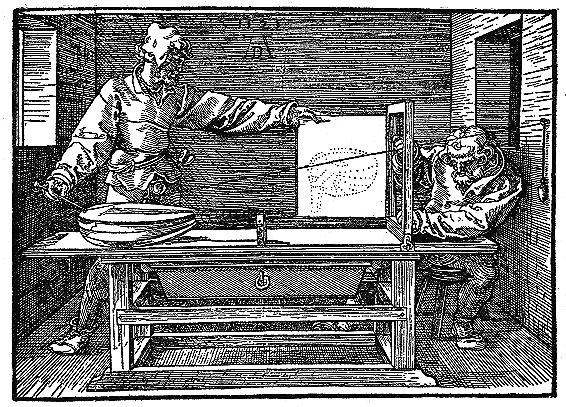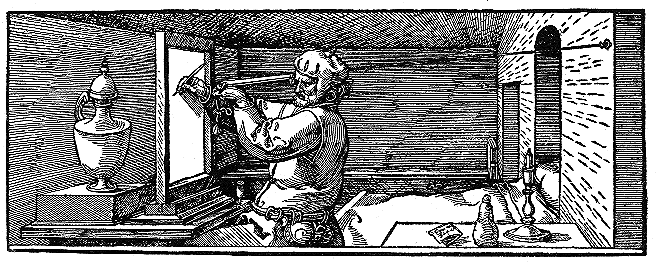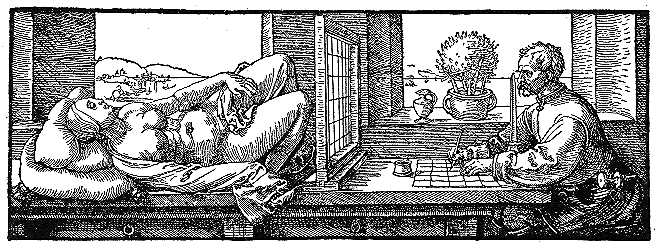# Perspective projection by Durer

Continuing my quest to relate everything to Computer Graphics, here're examples of the famous artist Albrecht Durer engraving himself doing perspective projections in 1525. They are from Hitachi's Viewseum, pictures 195, 196, and 197.Durer's Pictures for Geometry 2Durer's Pictures for Geometry 3Durer's Pictures for Geometry 4

Unfortunately, the Viewseum seems to have vanished; luckily I saved those local copies.

# Homogeneous Coordinates

## Motivation

1. Some common transformations are translation, scaling, rotation, projection.
2. To translate, you add. Translating p=(1,2,3)' by d=(4,5,6)': q=p+d
{$\begin{pmatrix}1\\2\\3\end{pmatrix} + \begin{pmatrix}4\\5\\6\end{pmatrix} = \begin{pmatrix}5\\7\\9\end{pmatrix}$}
3. Rotation and scaling are matrix multiplies:
{$R = \begin{pmatrix} .8&-.6&0\\.6&.8&0\\0&0&1\end{pmatrix}$}
{$S = \begin{pmatrix} 2&0&0\\ 0 &3&0\\0&0&4\end{pmatrix}$}
Then {$Rp$} and {$Sp$} rotate and scale p respectively.
4. Homogeneous coords make a translation or projection into a matrix multiply, like the others.
If {$p_h$} is the homogeneous form of {$p$} then {$T_h$} is a homogeneous matrix for the translation and {$T_h p_h$} translates {$p$}.
5. Now, several successive transformations can be combined into one matrix, which is then applied to the points in the object.
If you want to apply these transformations in order: {$M_1$}, {$M_2$}, {$M_3$}, {$M_4$} to an object with many points, then first compute {$M = M_4 M_3 M_2 M_1$} and then apply {$M$} to all the points. This is faster.

## Definition

1. In 3D, the homogeneous point {$(x_h, y_h, z_h, w)$} corresponds to the Cartesian point {$(x_h/w, y_h/w, z_h/w)$}
2. There are an infinite number of homogeneous ways to represent each Cartesian point. E.g., (1,1,1,1), (2,2,2,2) and (3,3,3,3) all correspond to the Cartesian point (1,1,1).
3. For finite points, w is not 0.

1. Translation is a matrix multiply. To translate by {$(d_x,d_y,d_z)$} multiply by this matrix: {$$\left( \begin{array}{cccc} 1 &0&0&d_x\\ 0&1&0&d_y\\ 0&0&1&d_z \\ 0&0&0&1 \end{array} \right)$$} For example, translate by (1,2,3): {$$M = \left(\begin{array}{cccc} 1 & 0 & 0 & 1\\ 0 & 1 & 0 & 2\\ 0 & 0 & 1 & 3\\ 0 & 0 & 0 & 1\end{array} \right)$$}
2. To translate p=(5,5,5), make it homogeneous: (5,5,5,1) and compute
3. {$$\begin{pmatrix} 6\\7\\8\\1\end{pmatrix} = \left(\begin{array}{cccc} 1 & 0 & 0 & 1\\ 0 & 1 & 0 & 2\\ 0 & 0 & 1 & 3\\ 0 & 0 & 0 & 1\end{array} \right) \begin{pmatrix}5\\5\\5\\1\end{pmatrix}$$}
4. (10,10,10,2) is the same homogeneous point, and gives the same Cartesian answer:
{$$\begin{pmatrix} 12\\14 \\16\\2\end{pmatrix} = \left(\begin{array}{cccc} 1 & 0 & 0 & 1\\ 0 & 1 & 0 & 2\\ 0 & 0 & 1 & 3\\ 0 & 0 & 0 & 1\end{array} \right) \begin{pmatrix}10\\10\\10\\2\end{pmatrix}$$}
5. Scaling the homogeneous matrix also works: {$$\begin{pmatrix} 12\\14 \\16\\2\end{pmatrix} = \left(\begin{array}{cccc} 2 & 0 & 0 & 2 \\ 0 & 2 & 0 & 4 \\ 0 & 0 & 2 & 6 \\ 0 & 0 & 0 & 2 \end{array} \right) \begin{pmatrix}5\\5\\5\\1\end{pmatrix}$$}
6. Scaling both the homogeneous point and matrix also works: {$$\begin{pmatrix} 24\\28 \\32\\ \textbf{4} \end{pmatrix} = \left(\begin{array}{cccc} 2 & 0 & 0 & 2 \\ 0 & 2 & 0 & 4 \\ 0 & 0 & 2 & 6 \\ 0 & 0 & 0 & 2 \end{array} \right) \begin{pmatrix}10\\10\\10\\2\end{pmatrix}$$}
(4 is a correction.)
7. You can still do the old matrix multiplies, like rotations, as homogeneous matrix multiplies. For example, rotate around Z axis by an angle whose cosine is 0.6: {$$R = \left(\begin{array}{rrrr} .6 & -.8 & 0 & 0 \\ .8 & .6 & 0 & 0 \\ 0 & 0 & 1 & 0 \\ 0 & 0 & 0 & 1 \end{array} \right)$$}
{$$R_2 = \left(\begin{array}{rrrr} 6 & -8 & 0 & 0 \\ 8 & 6 & 0 & 0 \\ 0 & 0 & 10 & 0 \\ 0 & 0 & 0 & 10 \end{array} \right)$$} gives the same Cartesian result.
(This was corrected by changing 60 and 80 to 6 and 8.)
8. Projection is a matrix multiply. To project onto the viewplane z=1 with the center at (0,0,0), the equations are thus: {$$\begin{eqnarray} x' &=& x/z\\ y' &=& y/z\\ z' &=& 1 \end{eqnarray}$$}
This is the same as multiplying by this matrix: {$$\left( \begin{array}{cccc} 1 &0&0&0\\ 0&1&0&0\\ 0&0&1&0 \\ 0&0&1&0 \end{array} \right)$$}
9. To project onto the viewplane z=10 with the center at (0,0,0), the equations are thus: {$$\begin{eqnarray} x' &=& 10 x/z\\ y' &=& 10 y/z\\ z' &=& 10 \end{eqnarray}$$}
This is the same as multiplying by this matrix: {$$\left( \begin{array}{cccc} 10 &0&0&0\\ 0&10 &0&0\\ 0&0&10 &0 \\ 0&0&1&0 \end{array} \right)$$}
10. To project onto the viewplane x=1 with the center at (0,0,0), the equations are thus: {$$\begin{eqnarray} x' &=& 1\\ y' &=& y/x\\ z' &=& z/x \end{eqnarray}$$}
This is the same as multiplying by this matrix: {$$\left( \begin{array}{cccc} 1 &0&0&0\\ 0&1&0&0\\ 0&0&1&0 \\ 1&0&0&0 \end{array} \right)$$}
11. Points at infinity can be represented. (1,2,3,0) is the infinite point at the end of the line from the origin thru Cartesian (1,2,3).
12. Therefore, a parallel projection has the same form as a perspective projection, with an infinite center of projection.
13. In 2-D, every pair of lines intersects. Two parallel lines intersect at infinity. What is the intersection of x=0 and x=1? Write the equations homogeneously thus: {$$\begin{eqnarray} 1x + 0y + 0w = 0\\1x + 0y - 1w = 0\end{eqnarray}$$} (All homogeneous equations have 0 as the constant.)
The solution is x=0, w=0, y=anything. The lines intersect at (0,1,0). Note that (0,2,0), (0,3,0), etc, are the same point.
14. Every two different points have one line thru them. If both points are infinite, this is the line at infinity, which contains all infinite points.
15. Computer aided design uses homogeneous points with w being the weight that a point has when approximating a curve near it. In homogeneous 2D, (1,1,1) and (2,2,2) are the same point, but using (2,2,2) will make the approximating curve come closer.
16. Suppose we want to represent a circle parametrically. Using Cartesian coordinates, there is no way to do this exactly with polynomials. However, here is an exact homogeneous rep: {$$\begin{eqnarray} \;x_h = t^2-1\\y_h = 2t \\w = t^2+1 \end{eqnarray}$$} You can see that is true since {$$x = \frac{x_h}{w} = \frac{t^2-1}{t^2+1}$$} {$$y = \frac{y_h}{w} = \frac{2t}{t^2+1}$$} and then {$$x^2+y^2 = \frac{(t^2-1)^2+(2t)^2}{(t^2+1)^2} =1$$}

## More Projection Matrices

Here are two more examples of how simple homogeneous coordinates make projections.

First, assume that the view plane is {$z=0$}, and the center of projection is at {$(0,0,-d), \, d>0$}. Using similar triangles, things scale by {$\frac{d}{z+d}$}. In Cartesian terms,{$$x' = x\left(\frac{d}{z+d}\right)$$} {$$y' = y\left(\frac{d}{z+d}\right)$$} {$$z' = 0$$}

In homogeneous terms, this would be

{$$x' = x$$} {$$y' = y$$} {$$z' = 0$$} {$$w' = \frac{z}{d} + w$$}

This is the same as multiplying by this matrix:

{$$\left( \begin{array}{cccc} 1 &0&0&0\\ 0&1&0&0\\ 0&0&0&0 \\ 0&0&1/d&1 \end{array} \right)$$}

In the limit as {$d\rightarrow\infty$}, and this becomes a parallel projection, we get in Cartesian terms,

{$$x' = x$$} {$$y' = y$$} {$$z' = 0$$}

In homogeneous terms, this would be

{$$x' = x$$} {$$y' = y$$} {$$z' = 0$$} {$$w' = w$$}

This is the same as multiplying by this matrix:

{$$\left( \begin{array}{cccc} 1 &0&0&0\\ 0&1&0&0\\ 0&0&0&0 \\ 0&0&0&1 \end{array} \right)$$}

Finally, here is a perspective projection onto an arbitrary view plane, with the center of projection at {$(0,0,0)$}. Let the equation of the plane be {$ax+by+cz=1$}. The point {$(x,y,z)$} will project to {$(x',y',z')$} with {$ax'+by'+cz'=1$}, so its 3 components will be scaled by {$1/(ax+by+cz)$}.

We get in Cartesian terms,

{$$x' = \frac{x}{ax+by+cz},\,\,\,\,\, y' = \frac{y}{ax+by+cz},\,\,\,\,\, z' = \frac{z}{ax+by+cz}$$}

In homogeneous terms, this would be

{$$x' = x$$} {$$y' = y$$} {$$z' = z$$} {$$w' = ax+by+cz$$}

This is the same as multiplying by this matrix:

{$$\left( \begin{array}{cccc} 1 &0&0&0\\ 0&1&0&0\\ 0&0&1&0 \\ a&b&c&0 \end{array} \right)$$}

Notice how simple it is.